## y = 2x – 3 y = -x + 3 solve for x and y

Question

y = 2x – 3
y = -x + 3 solve for x and y

in progress 0
3 weeks 2021-08-27T06:36:02+00:00 1 Answers 0 views 0

1. We are given the system of equations: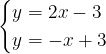Since both are y-isolated equation, we can combine them together.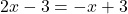Isolate and solve for x-term.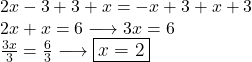Next, we find the value of y. Simply substitute x = 2 in one of these equations. The less coefficient values, the faster and better. I will substitute x = 2 in y = -x+3. You can substitute x = 2 in y = 2x-3 if you want but the result would be the same.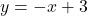Substitute x = 2 in the equation.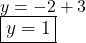Therefore – when x = 2, y = 1. We can write in coordinate point or ordered pair as (2,1) from (x,y).